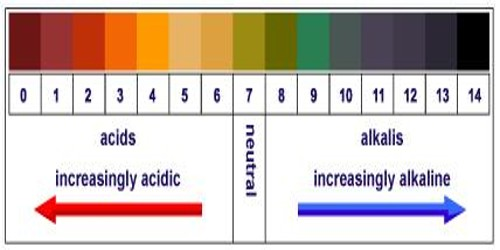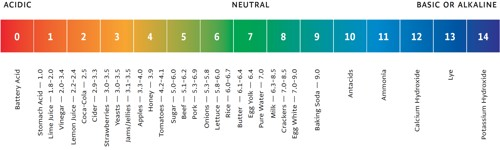# Determination of pH of a Solution

Determination of pH of a Solution

The change of potential with change in concentration of ions has been used in chemistry in many ways. Like all other electrodes, the potential of a hydrogen electrode which is reversible with respect to hydrogen ions changes with change in the concentration of H+ ions in accordance with the Nernst equation. Based on this fact methods have been devised to determine the pH of a solution from measurements of potentials of electrochemical cells. For this purpose a cell is set up with a standard electrode and an electrode containing the solution whose pH is to be measured. The standard electrode used for these measurements is usually a calomel electrode. The cell can be written as-

Pt│H2 (1 atm) (cH 1.0 molL-1)││Cl (1.0 molL-1)│Hg2Cl2(s)│HgUsing the Nernst equation the e.m.f of the cell at 25°C can be expressed as-

Ecell = EB – EL = Ecalomel – EH+

Ecell = Ecalomel –{EH+ + 0.0591/2 log [H+]2}

As at the hydrogen electrode the reaction taking place is-

2H+ + e ↔ H2

but, E0H+ = 0.00 V

Hence, Ecell = Ecalomel – 0.0591 log [H+]2 = Ecalomel + 0.0591 pH

or, (Ecell – Ecalomel) = 0.0591 pH

Therefore, pH = (Ecell – Ecalomel) / 0.0591